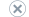# Use Aggregate Functions

Applies to:SQL Server Analysis ServicesAzure Analysis ServicesPower BI Premium

When a dimension is used to slice a measure, the measure is summarized along the hierarchies contained in that dimension. The summation behavior depends on the aggregate function specified for the measure. For most measures containing numeric data, the aggregate function is Sum. The value of the measure will sum to different amounts depending on which level of the hierarchy is active.

In Analysis Services, every measure that you create is backed by an aggregation function that determines the measure's operation. Predefined aggregation types include Sum, Min, Max, Count, Distinct Count, and several other more specialized functions. Alternatively, if you require aggregations based on complex or custom formulas, you can build an MDX calculation in lieu of using a prebuilt aggregation function. For example, if you want to define a measure for a percentage value, you would do that in MDX, using a calculated measure. See CREATE MEMBER Statement (MDX).

Measures that are created via the Cube Wizard are assigned an aggregation type as part of the measure definition. The aggregation type is always Sum, assuming the source column contains numeric data. Sum is assigned regardless of the source column's data type. For example, if you used the Cube Wizard to create measures, and you pulled in all columns from a fact table, you will notice that all of the resulting measures have an aggregation of Sum, even if the source is a date time column. Always review the pre-assigned aggregation methods for measures created via the wizard to make sure the aggregation function is suitable.

You can assign or change the aggregation method in the either the cube definition, via SQL Server Data Tools - Business Intelligence, or via MDX. See Create Measures and Measure Groups in Multidimensional Models or Aggregate (MDX) for further instructions.

## Aggregate Functions

SQL Server Analysis Services provides functions to aggregate measures along the dimensions that are contained in measure groups. The additivity of an aggregation function determines how the measure is aggregated across all the dimensions in the cube. Aggregation functions fall into three levels of additivity:

An additive measure, also called a fully additive measure, can be aggregated along all the dimensions that are included in the measure group that contains the measure, without restriction.

A semiadditive measure can be aggregated along some, but not all, dimensions that are included in the measure group that contains the measure. For example, a measure that represents the quantity available for inventory can be aggregated along a geography dimension to produce a total quantity available for all warehouses, but the measure cannot be aggregated along a time dimension because the measure represents a periodic snapshot of quantities available. Aggregating such a measure along a time dimension would produce incorrect results. See Define Semiadditive Behavior for details.

A nonadditive measure cannot be aggregated along any dimension in the measure group that contains the measure. Instead, the measure must be individually calculated for each cell in the cube that represents the measure. For example, a calculated measure that returns a percentage, such as profit margin, cannot be aggregated from the percentage values of child members in any dimension.

The following table lists the aggregation functions in SQL Server Analysis Services, and describes both the additivity and expected output of the function.

Sum Additive Calculates the sum of values for all child members. This is the default aggregation function.
Count Additive Retrieves the count of all child members.
Min Semiadditive Retrieves the lowest value for all child members.
Max Semiadditive Retrieves the highest value for all child members.
DistinctCount Nonadditive Retrieves the count of all unique child members. For more details, see About Distinct Count Measures in the next section.
None Nonadditive No aggregation is performed, and all values for leaf and nonleaf members in a dimension are supplied directly from the fact table for the measure group that contains the measure. If no value can be read from the fact table for a member, the value for that member is set to null.
ByAccount Semiadditive Calculates the aggregation according to the aggregation function assigned to the account type for a member in an account dimension. If no account type dimension exists in the measure group, treated as the None aggregation function.

AverageOfChildren Semiadditive Calculates the average of values for all non-empty child members.
FirstChild Semiadditive Retrieves the value of the first child member.
LastChild Semiadditive Retrieves the value of the last child member.
FirstNonEmpty Semiadditive Retrieves the value of the first non-empty child member.
LastNonEmpty Semiadditive Retrieves the value of the last non-empty child member.